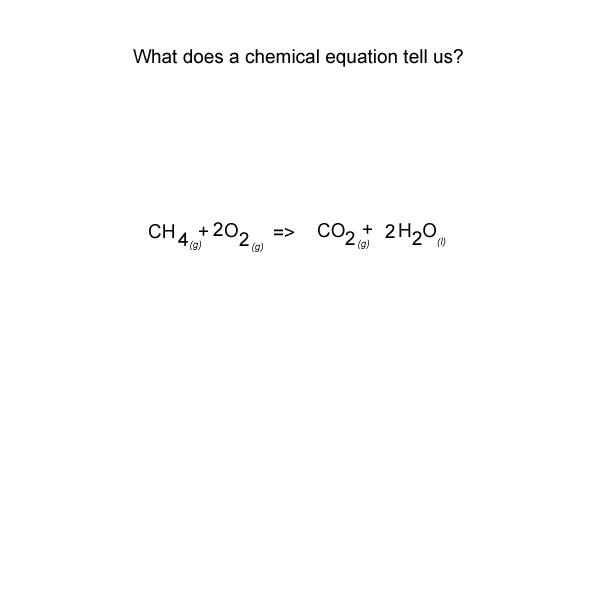Introduction to balanced chemical equations. A chemical equation is similar to a recipe. It states the ingredients (reactants) and the proportion of each ingredient used. It also gives the amount of product formed. Look at the animation below.See the video of the animation above. 1) What does the Law of Conservation of Mass state? mass can be created and destroyed during chemical reactions mass cannot be created and destroyed during chemical reactions mass is converted into energy during chemical reactions every chemical reaction can be written as a chemical equation if it involves converting mass into energy 2) A balanced chemical equation does not show the chemical formula of the reactants and products the size of the reactant particles or the size of the product particles. the ratio in which the reactants combine to form the products the states of the reactants and products 3) What must be the same on each side of a balanced chemical equation? the states of the reactants and products the number of atoms of each element the formula of the reactants and products 4) Consider the equation below. Ethene burns in oxygen to produce carbon dioxide and water according to the equation below. C2H4(g) + 3O2(g) => 2CO2(g) + 2H2O(l) a) How many carbon atoms are on the left of the equation? 4 5 2 3 b) Does this equation obey the Law of Conservation of Mass? True False c) Why the number of molecules on each side are the same. the number of atoms of each element are the same on both sides more oxygen atoms appear on the reactants side than on the products side. d) Each molecule of ethene has 3 4 5 2 carbon atoms and 3 4 5 2 atoms of hydrogen. e) Each molecule of oxygen is made of 3 4 5 2 atoms of oxygen f) When 5 molecule/s of ethene react with 3 4 15 12 molecules of oxygen to form 3 2 15 12 10 molecules of carbon dioxide and 3 2 15 12 10 of water. g) The water formed is in the gas liquid solid state. h) For every two molecules of ethene that react, 3 4 5 2 molecules of water form. i) If 6 molecules of oxygen react, how many molecules of carbon dioxide will form? 3 4 5 2 5) Consider the equation below. Sodium carbonate powder is placed in a solution of hydrochloric acid and reacts vigorously to produce carbon dioxide gas, liquid water and dissolved sodium chloride according to the equation below. Na2CO3(s) + 2HCl(aq) => CO2(g) + H2O(l) + 2NaCl(aq) a) How many oxygen atoms are on the left of the equation? 4 5 2 3 b) Does this equation obey the Law of Conservation of Mass? False True . Explain why. c) Each molecule of carbon dioxide has 5 1 3 4 carbon atoms and 4 5 2 3 atoms of oxygen . d) If 2 molecules of HCl reacted how many molecules of CO2 are produced? 5 1 3 4 e) If 4 molecules of HCl reacted how many molecules of CO2 are produced? 5 1 3 2 f) The salt (NaCl) formed is in the liquid solid aqueous gas state. g) For every one molecule of water that is produced, 5 2 3 1 molecules of HCl react.. h) If 6 molecules of HCl react, how many molecules of carbon dioxide will form? 4 5 2 3 6) Consider the chemical equation below. It shows nitrogen and hydrogen gases reacting to form ammonia gas. N2(g) + H2(g) => NH3(g) a) All the reactant particles are in the liquid solid aqueous gas state b) An ammonia molecule consists of one nitrogen and two hydrogen atoms one nitrogen and three hydrogen atoms three nitrogen and two hydrogen atoms one nitrogen and one hydrogen atom c) Explain why this chemical equation is not considered to be balanced? d) Select the appropriate prefixes that should be placed in front of each reactant and product in order to balance this equation. 5 2 3 1 N2(g) + 5 2 3 1 H2(g) => 5 2 3 1 NH3(g) e) In your balanced equation given in question d) above :         i.    how many ammonia molecules appear on the products side of the equation. 2 3 1 6 5         ii.   how many hydrogen atoms appear on the products side of the equation. 2 3 1 6 5 Solutions Continue